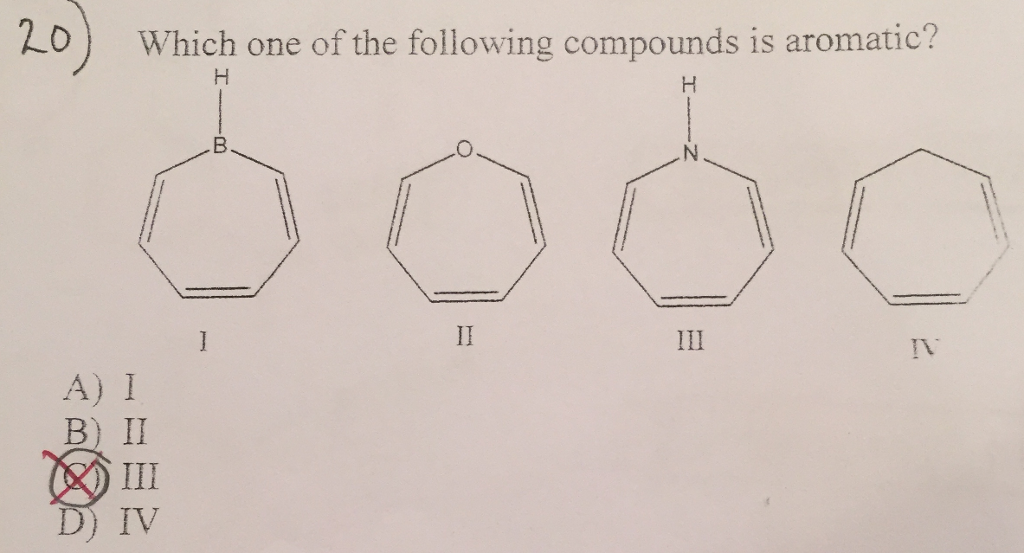# Which one of the following compounds is aromatic

ExplainWhich one of the following compounds is aromatic? A) I B) II C) III D) IV

The following are the rules for aromaticity:
a) The compound must be cyclic.
b) Every atom in the ring must be conjugated.
c) The compound must be planer.
d) The compound must have (4n + 2)π electrons where n = 0, 1, 2,
3…..
All the four compounds are cyclic. However, not all of these are
aromatic.
I is planer, cyclic and has (4n + 2)π = 6 electrons and hence n
= 1. Therefore, I is aromatic.
II is cyclic, planer and every atom is conjugated. It is
important to note that although there are two non-bonding electron
pairs over the O atom, not both the pairs are in the same plane as
the ring. Only one pair is in the same plane as the ring and the
other pair is perpendicular to the ring. The lone electron pair
which is in the plane of the ring is conjugated. However, we shall
have 8 electrons which violates the (4n + 2)π rule and hence II is
not aromatic.
III is planer and cyclic. All the atoms are conjugated; however
the molecule has 8 electrons (the N atom has only one non-bonding
electron pair in the same plane as the ring). The (4n + 2)π rule is
violated and hence, III is non-aromatic.
IV is cyclic and has 6 π electron which satisfy the (4n + 2)π
rule. However, the singly bonded C atom lies out of the plane and
breaks the planarity of the ring. Hence, IV is non-aromatic.